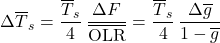# Analysis: Small Changes in Climate Variables

Note: This post is highly mathematical. This page references results from these other pages:

It can be useful to consider what happens when small changes are made to the climate system.

## Conservation of Energy

I’ll begin with the Conservation of Energy equation for a planet’s “climate zone.” This can be expressed as:

(1)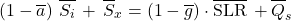(2)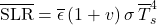The above equations are valid at all times. In particular, they are valid at times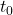and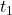. Suppose that, for each variable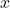, the change inbetween these two times is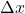so that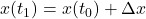.

## Small Changes

We can develop equations which are valid for small changes using the following procedure:

1. Write down the equations forand for, where the later values are expressed in terms of the value atplus the associated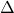value.
2. Multiply out the equations for, discarding all terms containing the product of two or morevalues. This procedure will be accurate in the limit of small delta values.
3. Subtract the equations forfrom the equations for.

The result of this procedure is the equations below:

(3)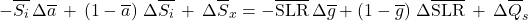(4)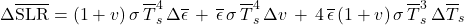(The values of each quantity other than the‘s is implicitly the value at time.)

## Formulas for Change in Surface Longwave Radiation flux

With some rearrangement, the above equations can be rewritten as:

(5)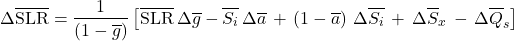(6)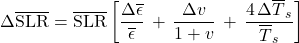Considering the first of the two equations above, we see that, for energy to be conserved, any small change in the energy fluxes on the right must be multiplied by a factor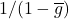to obtain the corresponding change in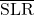. This might seem odd, but it reflects the fact that the energy conservation equation relates directly to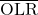, and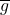is defined so that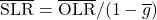. For Earth at present,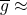0.40, so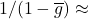1.67.

## Formula for Change in Mean Surface Temperature

Combining the last two equations above and solving for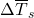, we find:

(7)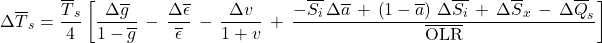This formula relates changes in different quantities, and is valid whenever changes are sufficiently small that each change is small in comparison to the value being changed. If changes are large, or precise work, one can instead use the exact formula for non-equilibrium mean surface temperature.

## Formula for Change in Mean Surface Brightness Temperature

If one is working with the mean global surface brightness temperature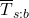the results are quite similar:

(8)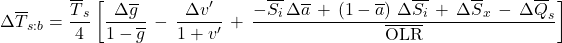The change in mean global surface temperatureis related to this by:

(9)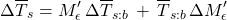## Greenhouse effect forcing

A “forcing” in the greenhouse effect is typically characterized by the change to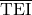when no other parameters are allowed to change. Given the above formula for “small changes”, one can infer that if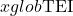changes by an amount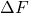, then this will be related to the change in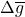by:

(10)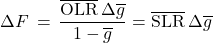## Simple response to forcing

If a forcing results in a change inby an amount, then the temperature subsequently changes to cancel out this imbalance, with no other variables changing, then one finds:

(11)